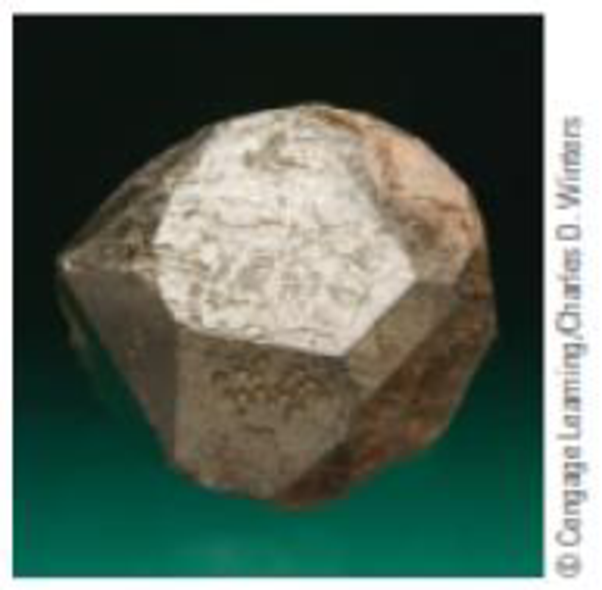Chapter 12, Problem 53GQ

Chapter
Section
Textbook Problem

Spinels are solids with the general formula AB2O4 (where A2+ and B3+ are metal cations of the same or different metals). The best-known example is common magnetite, Fe3O4 [which you can formulate as (Fe2+)(Fe3+)2O4]. Another example is the mineral often referred to as spinel, MgAl2O4.A crystal of the spinel MgAl2O4The oxide ions of spinels form a face-centered cubic lattice. In a normal spinel, cations occupy ⅛ of the tetrahedral sites and ½ of the octahedral sites. (a) In MgAl2O4, in what types of holes are the magnesium and aluminum ions found? (b) The mineral chromite has the formula FeCr2O4. What ions are involved, and in what types of holes are they found?

(a)

Interpretation Introduction

Interpretation:

Types of holes where magnesium and aluminum ions are found have to be identified.

Concept introduction:

Spinels:

The spinels are any of a class of minerals of general formulation AB2X4 which are cubic (isometric) crystal system.

Explanation

Mg2+ occupies 1/8 of 8 possible tetrahedral holes which formed by a planar triangle of atoms, with a fourth atom covering the indentation in the center

(b)

Interpretation Introduction

Interpretation:

The ion involved and the types of holes that are found in FeCr2O4 has to be identified.

Concept introduction:

Spinels:

The spinels are any of a class of minerals of general formulation AB2X4 which are cubic (isometric) crystal system.

Still sussing out bartleby?

Check out a sample textbook solution.

See a sample solution

The Solution to Your Study Problems

Bartleby provides explanations to thousands of textbook problems written by our experts, many with advanced degrees!

Get Started